﻿ 基于自组织网络的舰船通信系统研究
 舰船科学技术2022, Vol. 44Issue (14): 143-146    DOI: 10.3404/j.issn.1672-7649.2022.14.030PDF

Research on ship communication system based on self-organizing network
WANG Ying
Information Engineering Institute, Jiangsu Maritime Institute, Nanjing 211199, China
Abstract: This paper summarizes the self-organizing network technology, and emphatically analyzes the definition and characteristics of self-organizing network. This paper studies the capacity of ad hoc networks, focusing on the capacity of ad hoc networks in multi-channel structure. A time synchronization method for ad hoc networks is proposed. Finally, the simulation of ad hoc network is carried out to analyze the changes of data transmission in the network under the condition of different number of network terminal nodes.
Key words: warship     self organizing network     communication system
0 引　言

1 自组织网络技术 1.1 自组织网络定义及其特点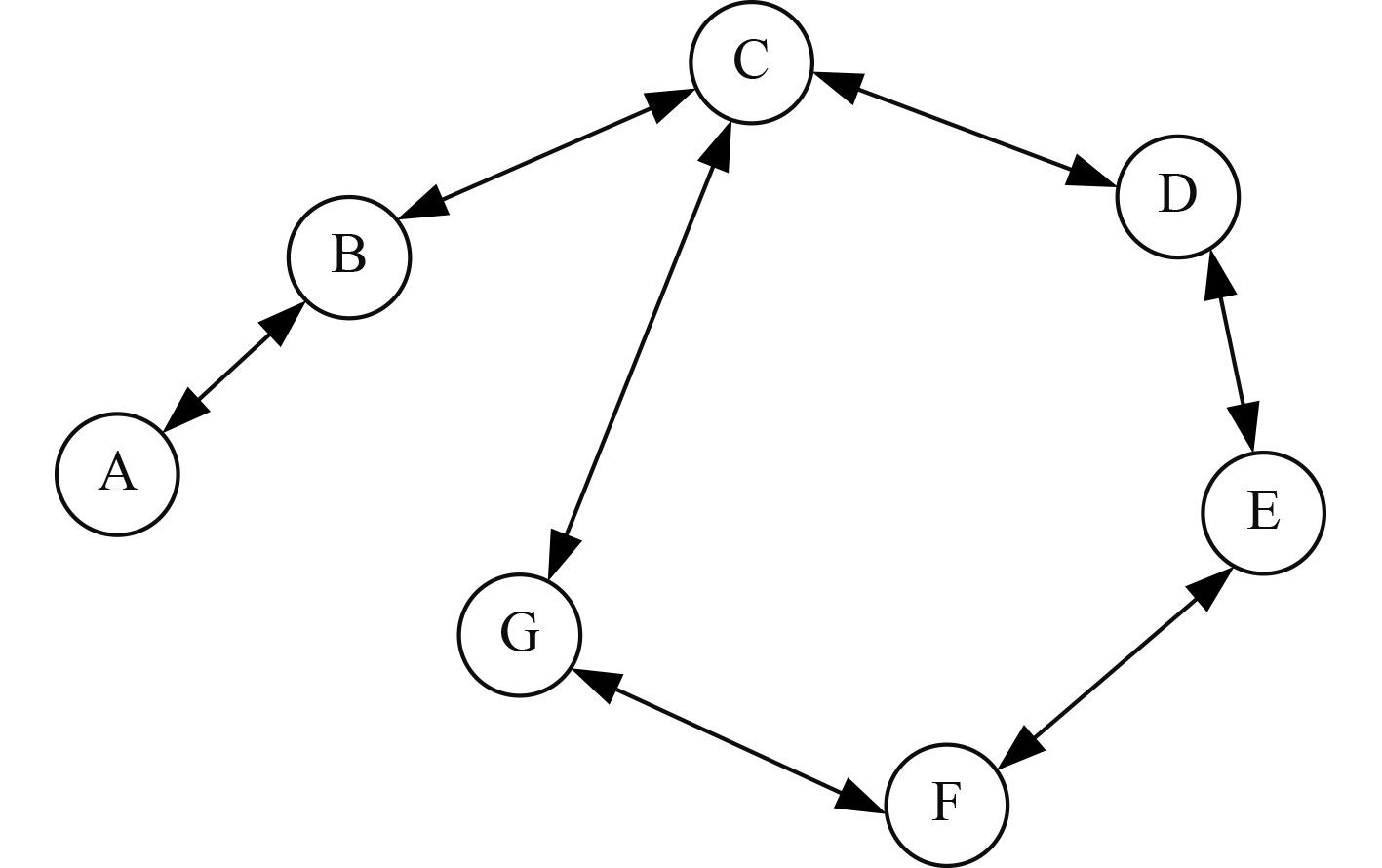图 1 自组织网络的基本结构 Fig. 1 Basic structure of ad hoc network

1.2 自组织网络的容量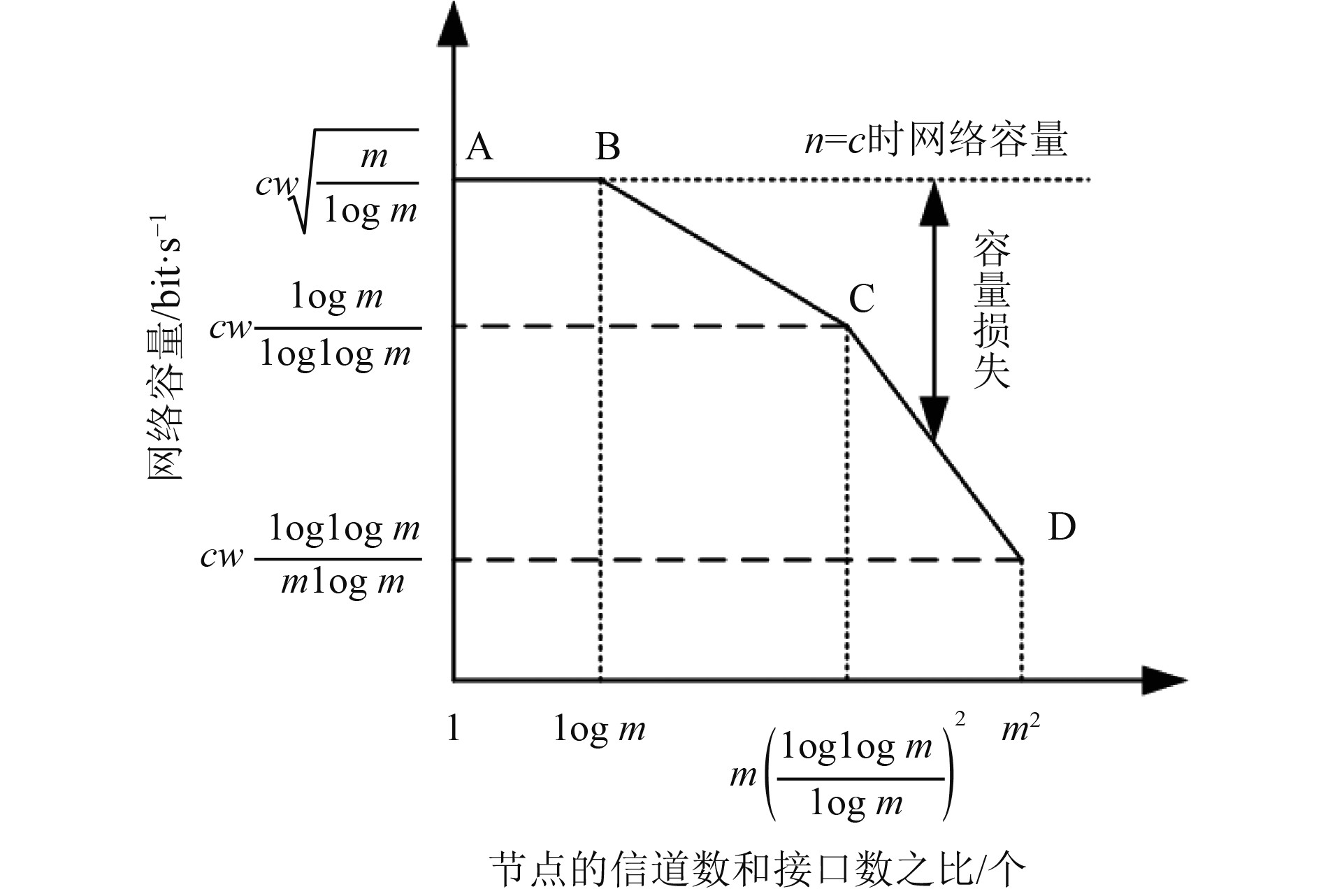图 2 信道数和接口数之比与吞吐量之间的关系 Fig. 2 Relationship between the ratio of channel number to interface number and throughput
1.3 自组织网络中的时间同步

 ${T_i}\left( t \right) = {f_i}t + {\theta _i}。$ (1.1)

 ${C_i}\left( t \right) = {\alpha _i}{T_i}\left( t \right) + {\beta _i} = {\alpha _i}{f_i}t + {\alpha _i}{\theta _i} + {\beta _i} = {\hat f_i}t + \hat \theta 。$ (1.2)

 $\bar C\left( t \right) = \bar ft + \bar \theta 。$ (1.3)

 ${C_i}\left( t \right) = F\left( {{{\bar C}_i}\left( t \right),{{\bar C}_j}\left( t \right)} \right)。$ (1.4)

 ${C_i}\left( t \right) = {\hat f_i}\bar C\left( t \right) + {\hat \theta _i}\left( t \right) 。$ (1.5)

 ${\hat f_i}\left( t \right) = {G_i}\left( {{{\bar C}_i}\left( t \right),{{\bar C}_j}\left( t \right)} \right)\text{，}$ (1.6)
 ${\hat \theta _i}\left( t \right) = {H_i}\left( {{{\bar C}_i}\left( t \right),{{\bar C}_j}\left( t \right)} \right)。$ (1.7)

 $\mathop {\lim }\limits_{x \to \infty } {C_i}\left( t \right) = \bar C\left( t \right) = \bar ft + \bar \theta 。$ (1.8)
2 船舶自组织网络通信系统仿真分析 2.1 仿真环境及性能指标

 $PDR = \frac{{{N_{{\rm{received}}}}}}{{{N_{{\rm{lost}}}} + {N_{{\rm{received}}}}}}\text{，}$ (2.1)
 $PLR = \frac{{{N_{{\rm{lost}}}}}}{{{N_{{\rm{lost}}}} + {N_{{\rm{received}}}}}}。$ (2.2)

 ${T_{{\rm{aver\_delay}}}} = \frac{1}{{{N_{{\rm{received}}}}}}\sum\limits_{i = 1}^{{N_{{\rm{received}}}}} {\left( {r{t_i} - s{t_i}} \right)} 。$ (2.3)

2.2 仿真结果分析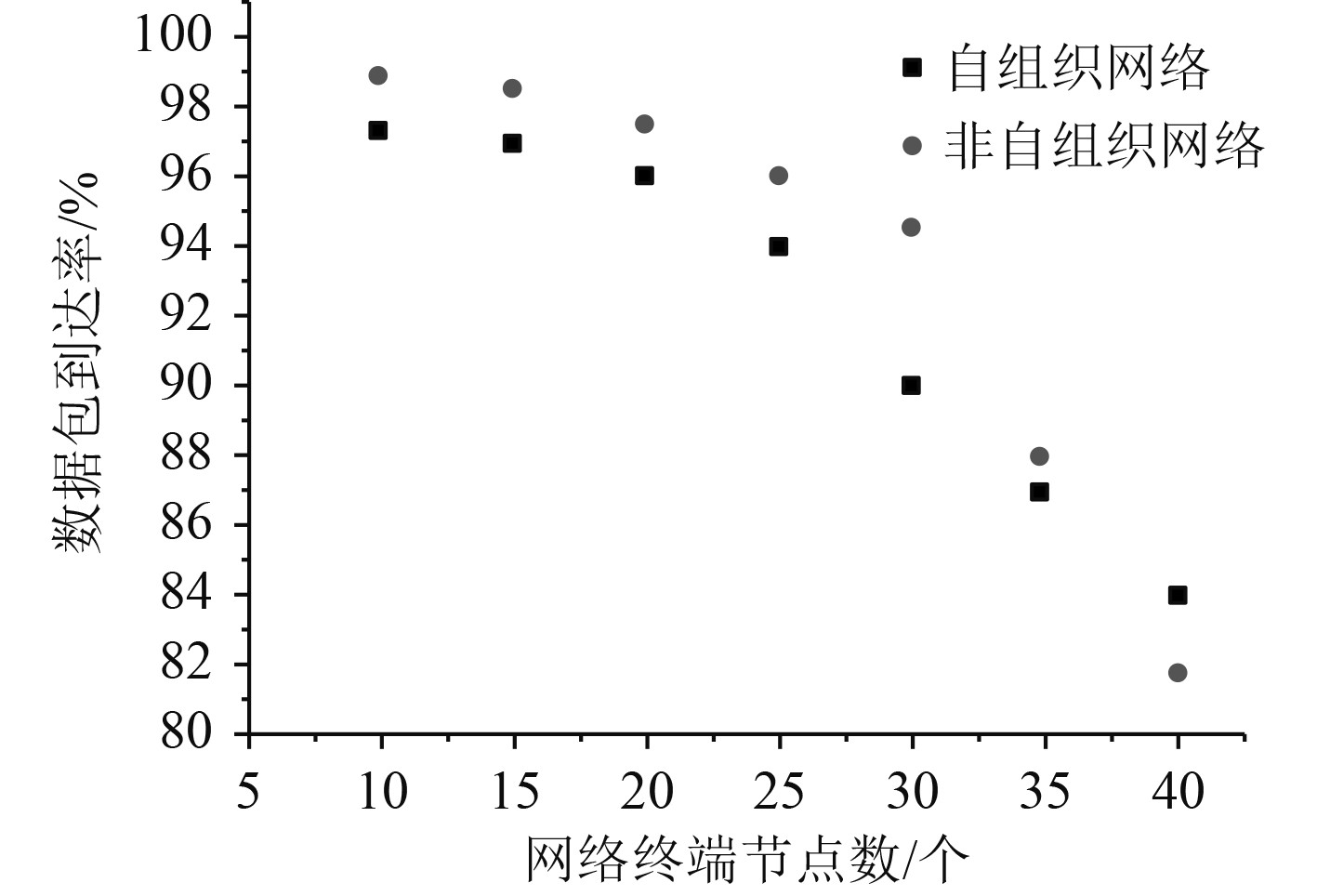图 3 数据包到达率和网络终端节点个数之间的关系 Fig. 3 Relationship between packet arrival rate and number of network terminal nodes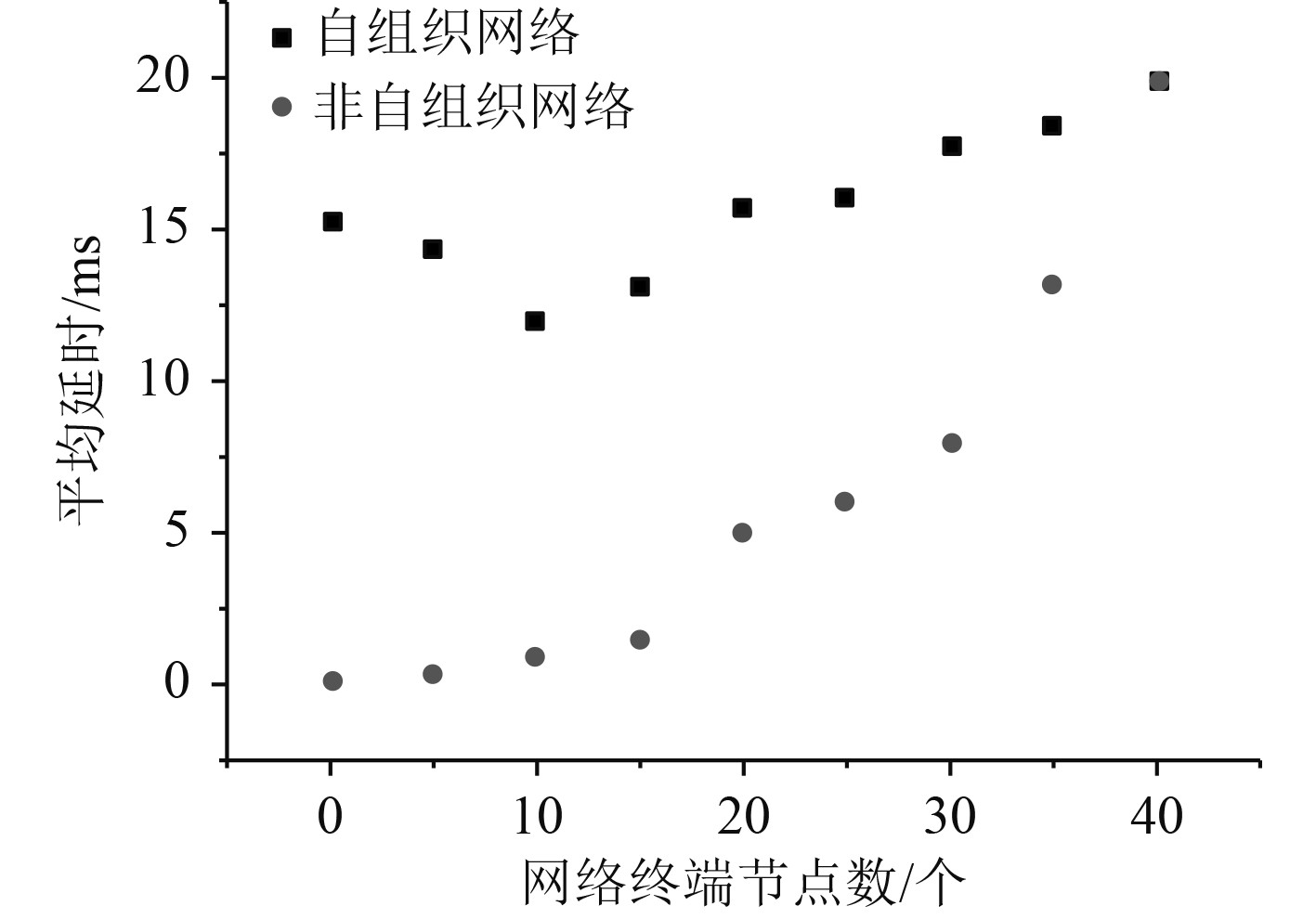图 4 平均到达时间和网络终端节点个数之间的关系 Fig. 4 Relationship between average arrival time and number of network terminal nodes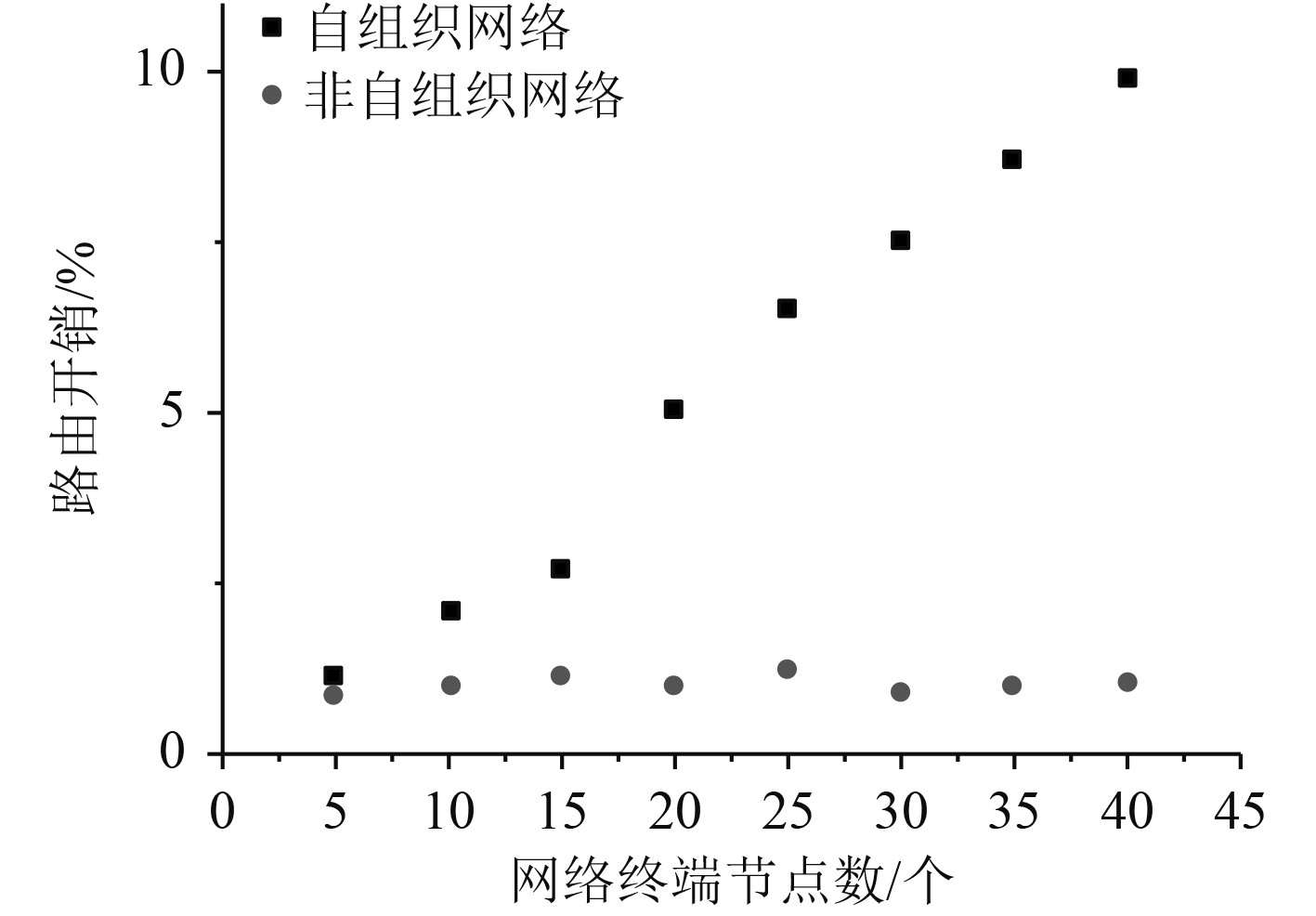图 5 路由开销和网络终端节点个数之间的关系 Fig. 5 Relationship between routing overhead and number of network terminal nodes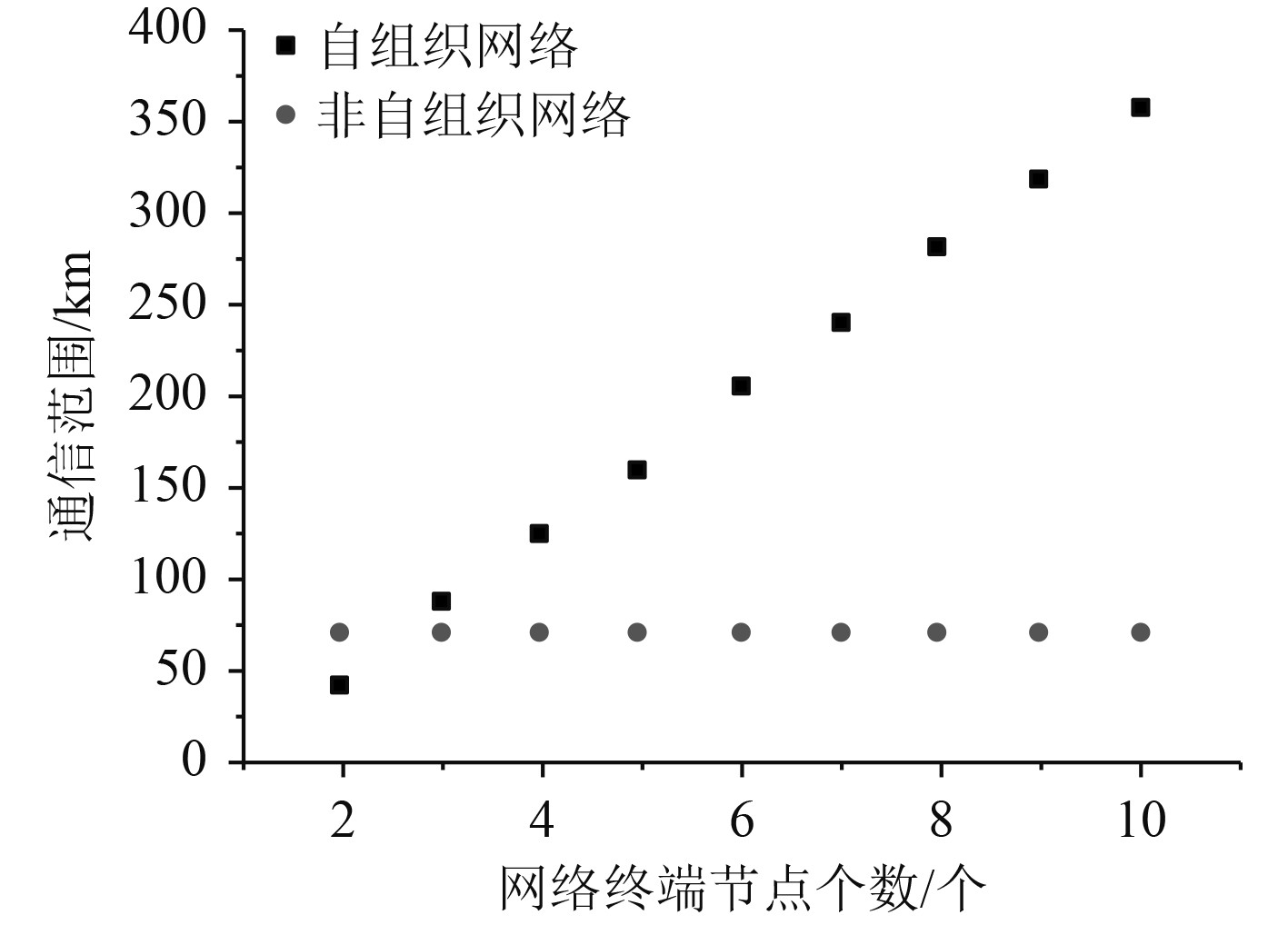图 6 网络通信范围和网络终端节点个数之间的关系 Fig. 6 Relationship between network communication range and number of network terminal nodes
3 结　语

  张淑华. 干扰抵消算法的船舶通信系统构建[J]. 舰船科学技术, 2020, 42(12): 139-141.  翟文学. 物联网环境下船舶通信系统重复不完整数据自动挖掘方法[J]. 舰船科学技术, 2021, 43(14): 127-129.  刘芳, 胡立章, 王亚静等. 智能电网应急通道中无线自组织网络的接入技术研究[J]. 河北电力技术, 2017(36): 22-24.  贾欣歌, 计春雷等. 自组织网络路由协议及仿真技术研究[J]. 计算机应用与软件, 2015(32): 114-116. DOI:10.3969/j.issn.1000-386x.2015.08.027  葛晨, 孙宁, 肖广兵. 车载自组织网络中并发广播冲突研究[J]. 自动化仪表, 2022(43): 106-110.  吴亚联, 朱紫琦, 黄盟标, 等. 基于区块链的车载自组织网络群签名方案[J]. 吉林大学学报, 2022(52): 1161-1167.  刘朝霞, 杨少石, 张涛, 等. 一种面向多业务服务质量优化的飞行自组织网络路由协议[J]. 中国传媒大学学报, 2022(29): 19-26.  吕文红, 屈衍玺, 徐峰, 等. 车载自组织网络中AODV协议研究进展[J]. 山东科技大学学报, 2021(40): 105-115.